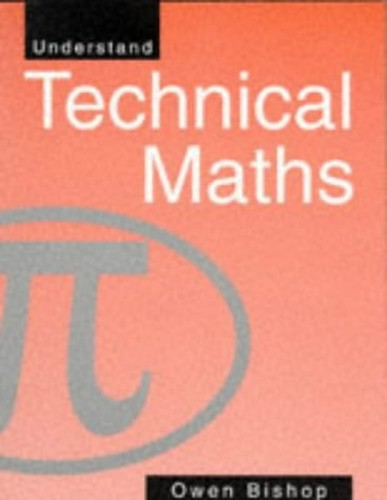Free Shipping on all orders in Australia
Over 7 million books in stock
We aim to be carbon neutral by 2022# Understand Technical Maths by O.N. Bishop

Condition - Good
\$36.99
Only 1 left

## Summary

This textbook is for beginners on technician engineering courses, using examples, exercises and suggestions for exploratory work. Unlike many textbooks, the maths is related to examples in practical engineering. The book is divided into four distinct parts.

## Understand Technical Maths Summary

### Understand Technical Maths by O.N. Bishop

This textbook is for beginners on technician engineering courses, using examples, exercises and suggestions for exploratory work. Unlike many textbooks, the maths is related to examples in practical engineering. The book is split into four parts, the first part looks at the maths essentials, fully explaining and consolidating elementary maths for the benefit of weak students. The second takes the student into the areas of maths which apply to most branches of technology while the third part introduces, and explains the more advanced aspects that are required in certain areas of technology. The final section looks at aspects of handling data and elements of statistics.

## Table of Contents

Plus and minus; times and divide; fractions; letters for numbers; lines, angles and shapes; areas and volumes; graphs; using essential maths; factors, ratios and proportions - indexes, logs; factors in algebra; handling equations and formulae; more about triangles; circles; more areas and volumes; working with graphs; using basic maths; simultaneous equations; using graphic calculators; polar graphs; vectors; complex numbers; rates of change; equations for summing; planning projects with maths; using spreadsheets; analyzing numbers; using math topics; collecting data; displaying data; more about spreadsheets; analyzing data; quality control.

## Additional information

GOR003361720
Understand Technical Maths by O.N. Bishop
Used - Good
Paperback
Taylor & Francis Ltd
1994-08-15
328
0750619554
9780750619554
N/A
Book picture is for illustrative purposes only, actual binding, cover or edition may vary.
This is a used book - there is no escaping the fact it has been read by someone else and it will show signs of wear and previous use. Overall we expect it to be in very good condition, but if you are not entirely satisfied please get in touch with us